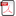### The New Bivariate Conway-Maxwell-Poisson Distribution Obtained by the Crossing Method

•  Rufin Bidounga
•  Evrand Giles Brunel Mandangui Maloumbi
•  Réolie Foxie Mizélé Kitoti
•  Dominique Mizère

#### Abstract

Kimberly et al. had proposed in 2016 a bivariate function as a bivariate Conway-Maxwell-Poisson distribution (COM-Poisson) using the generalized bivariate Poisson distribution and the probability generating functions of the follow distributions: bivariate bernoulli, bivariate Poisson, bivariate geometric and bivariate binomial. By examining this paper we have shown that this bivariate function is constant and it double series is divergent, when it should have been 1. To overcome this deadlock, we propose a new bivariate Conway-Maxwell-Poisson distribution which is definetely a probability distribution based on the crossing method, method highlighted by Elion et al. in 2016 and revisited by Batsindila et al. and Mandangui et al. in 2019. And this is the purpose of this paper. To this bivariate distribution is attached two generalized linear models (GLM) whose resolution allows us to highlight, not only the independence between the variables forming the couple, but also the effect of the factors (or predictors) on these variables. The resulting correlation is negative, zero or positive depending on the values of a parameter; in particular for the bivariate Poisson distribution according to Berkhout and Plug. A simulation of data will be given at the end of the article to illustrate the model.

• Full Text:PDF
• DOI:10.5539/ijsp.v9n6p1This work is licensed under a Creative Commons Attribution 4.0 License.
• ISSN(Print): 1927-7032
• ISSN(Online): 1927-7040
• Started: 2012
• Frequency: bimonthly

### Journal Metrics

• h-index (December 2020): 18
• i10-index (December 2020): 36
• h5-index (December 2020): N/A
• h5-median(December 2020): N/A